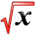# Applications of the Definite IntegralThis article is part of the MathHelp Tutoring Wiki

Future Value of a continuous income stream Formula :

N0 K$e^{r(N-t)}$dt
K = dollars per year
N = years at interest rate r
r = interest rate

Example 1 :

At an annual rate of \$1000, money is being deposited into a savings account everyday. The savings account pays 6% interest that is compounded continuously. What is approximately the amount of money in the account after 5 years?
Solution :
50$1000e^{0.06(5-t)}$dt
=${\frac {1000}{-0.06}}e^{0.06(5-t)}$|50
=${\frac {1000}{-0.06}}(1-e^{0.3})$= approximately = 5831